# Custom Axis Labels

EGRET was designed for water-quality and streamflow exploration. The units for streamflow are stored within the EGRET object (eList) in m^3/s. The units for the water-quality measurements are stored in mg/l. With these units in mind, all the plots and tables offer the user simple alternatives for display. This article will describe how to use the different options, and how to create your own custom labels.

# Streamflow

Streamflow values are stored as m^3/s. For plots and tables that display discharge data, there is a user-argument to the functions called qUnit. This input can be a numeric between 1 and 4, or it can be one of the following text: “cfs”, “cms”, “thousandCfs”, or “thousandCms”. The numeric that lines up with the text can be seen with the printqUnitCheatSheet function:

printqUnitCheatSheet()
## The following codes apply to the qUnit list:
## 1 =  cfs  ( Cubic Feet per Second )
## 2 =  cms  ( Cubic Meters per Second )
## 3 =  thousandCfs  ( Thousand Cubic Feet per Second )
## 4 =  thousandCms  ( Thousand Cubic Meters per Second )

Here is an example of using the default (qUnit = 2 which corresonds to “cms”), compared to using qUnit = 1 (corresponding to “cfs”):

eList <- Choptank_eList
plotConcQ(eList)
plotConcQ(eList, qUnit = 1)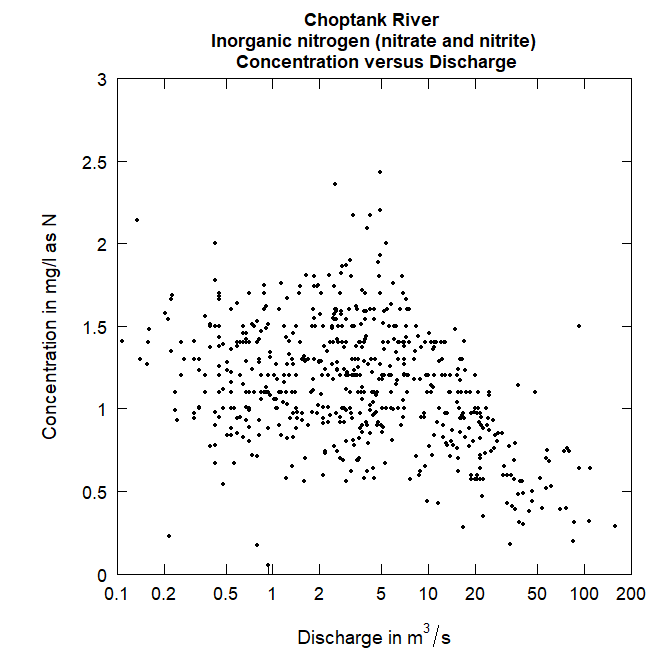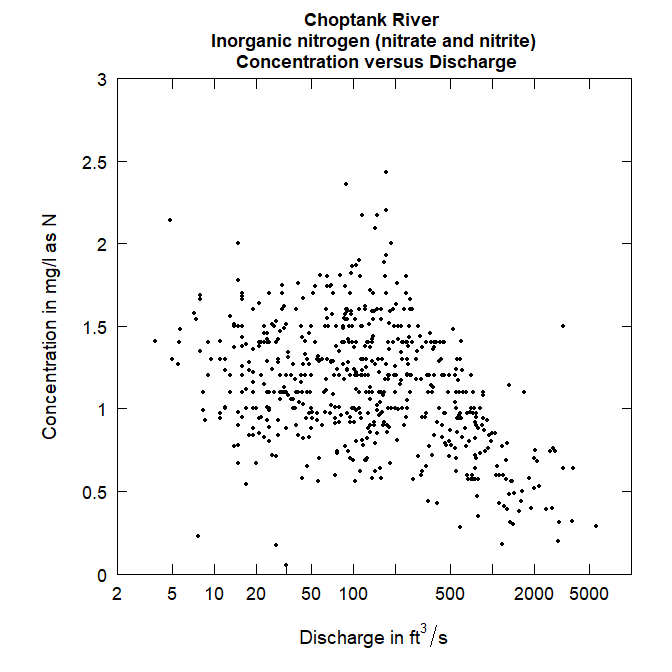Plots of discharge with different units.

## Custom Units

There could be a scenario where EGRET analysis is not done using a streamflow value. For example, let’s say we want to use precipitation as a substitution for discharge. A very important point here is that if you use data that are not designed for the EGRET software, it will probably not make sense to use the flux calculations. But, there are places where EGRET graphs and tables will be interesting using this alternative data.

Here is an example of how to make a custom qUnit object. It is not as simple as adding a single line of text. This is because the software needs to know how to create nice labels for a variety of different kind of plots, and if there is a conversion factor, it would need to know that conversion factor. This example shows how to make a custom qUnit for precipitation with millimeters as the unit:

qConst_precip <- new("qUnit",
qShortName = "   mm  ",
qUnitFactor = 1,
qUnitName = "Millimeter",
qUnitExpress = expression(paste("Precipitation in ",mm)),
qUnitTiny = expression(paste("Precipitation ", "(", mm, ")")),
shortCode = 1,
unitUSGS = "Precipitation, in mm",
prefix = "Precipitation")

Each “slot” in this qUnit object is required, and the qShortName, qUnitName, unitUSGS, and prefix must be characters. The qUnitFactor must be a numeric (this would be the conversion factor that the data gets multiplied by). qUnitExpress and qUnitTiny are “expressions” which allows the text to be formatted. To use this custom unit:

plotConcQ(eList, qUnit = qConst_precip)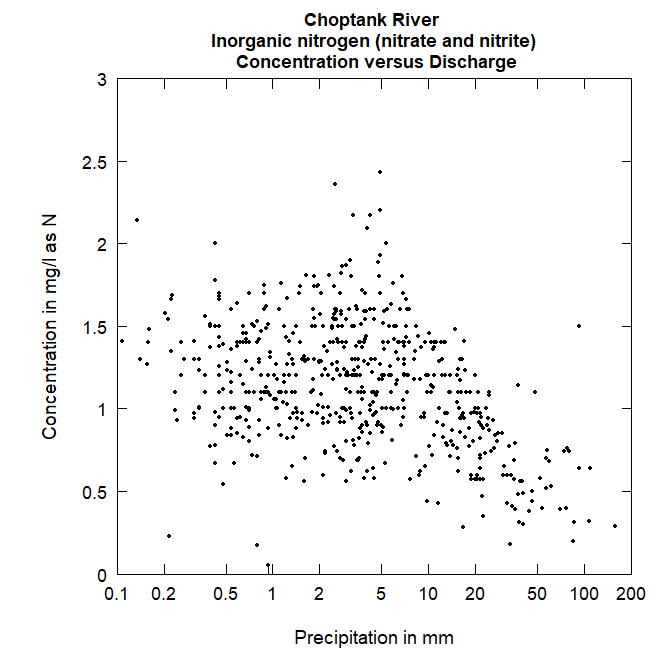Plot with custom axis, using precipitation instead of discharge.

## Data input

Discharge data in units other than m^3/s can be used as input in EGRET, but that data will be converted to m^3/s with the qUnit argument when the eList is created. So if you have data that is coming in that is in cubic feet per second, you could use the readDailyUser function, and specify qUnit = 1. This will take your cfs data, and store it properly as cms.

# Concentration

Concentration values are stored as mg/l. This is the only option that comes by default in EGRET. However, you can change the unit display using the eList$INFO$param.units (there are not conversion options however). You can also create a custom concUnit as shown below:

eList$INFO$param.units <- "ng"

deposition <- new("concUnit",
longPrefix = "Deposition",
shortPrefix = "Dep")

plotConcQ(eList,
qUnit = qConst_precip,
concLab = deposition)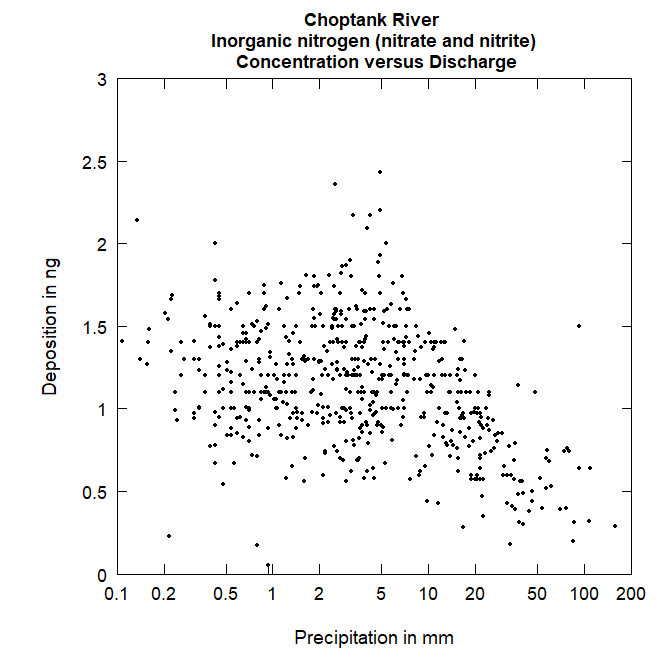# Flux

There are a variety of units of flux that can be used. For plots and tables that display flux data, there is a user-argument to the functions called fluxUnit. This input can be a numeric between 1 and 13, or it can be one of the following text:

names(fluxConst)
##   "poundsDay"        "tonsDay"          "kgDay"            "thousandKgDay"
##   "tonsYear"         "thousandTonsYear" "millionTonsYear"  "thousandKgYear"
##   "millionKgYear"    "billionKgYear"    "thousandTonsDay"  "millionKgDay"
##  "kgYear"

The numeric that lines up with the text can be seen with the printFluxUnitCheatSheet function:

printFluxUnitCheatSheet()
## The following codes apply to the fluxUnit list:
## 1 =  poundsDay  ( pounds/day )
## 2 =  tonsDay  ( tons/day )
## 3 =  kgDay  ( kg/day )
## 4 =  thousandKgDay  ( thousands of kg/day )
## 5 =  tonsYear  ( tons/year )
## 6 =  thousandTonsYear  ( thousands of tons/year )
## 7 =  millionTonsYear  ( millions of tons/year )
## 8 =  thousandKgYear  ( thousands of kg/year )
## 9 =  millionKgYear  ( millions of kg/year )
## 10 =  billionKgYear  ( billions of kg/year )
## 11 =  thousandTonsDay  ( thousands of tons/day )
## 12 =  millionKgDay  ( millions of kg/day )
## 13 =  kgYear  ( kg/year )

Here is a comparison of the default values and a specified flux unit.

plotFluxHist(eList)
plotFluxHist(eList, fluxUnit = 2)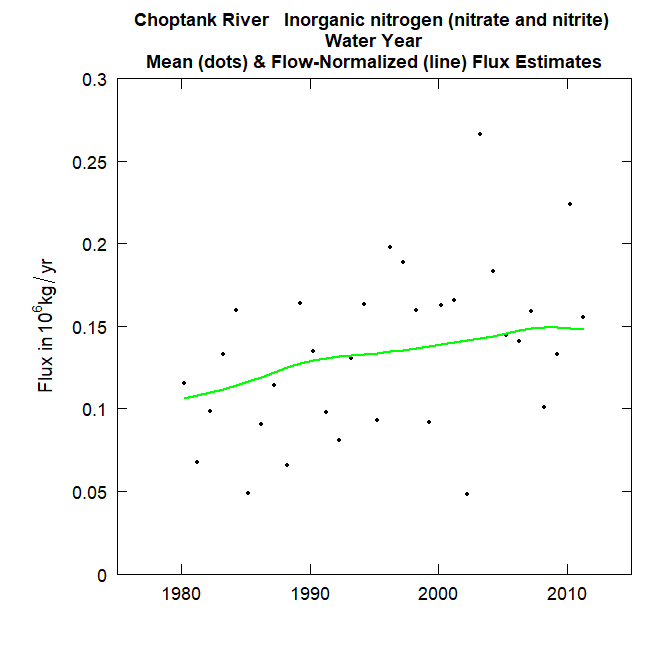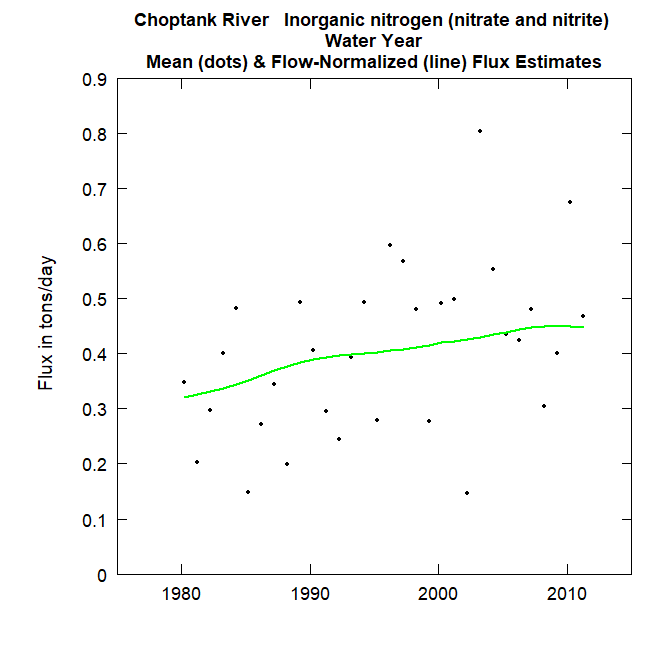Plots of flux with different units.

Here is an example of how to make a custom fluxUnit object. It is not as simple as adding a single line of text. This is because the software needs to know how to create nice labels for a variety of different kind of plots, and if there is a conversion factor, it would need to know that conversion factor. This example shows how to make a custom fluxUnit of grams per day:

gDay <- new("fluxUnit",
shortName = "    g/day   ",
unitFactor = 1000,
unitName = "g/day",
unitExpress = expression("Flux in g/day"),
unitExpressTiny = expression("Flux (g/day)"),
unitEstimate = expression("flux in g/year"),
unitEstimateTiny = expression("Est. flux in g/day"),
unitUSGS = "Flux, in grams per day",
shortCode = 14)

plotFluxHist(eList, fluxUnit = gDay)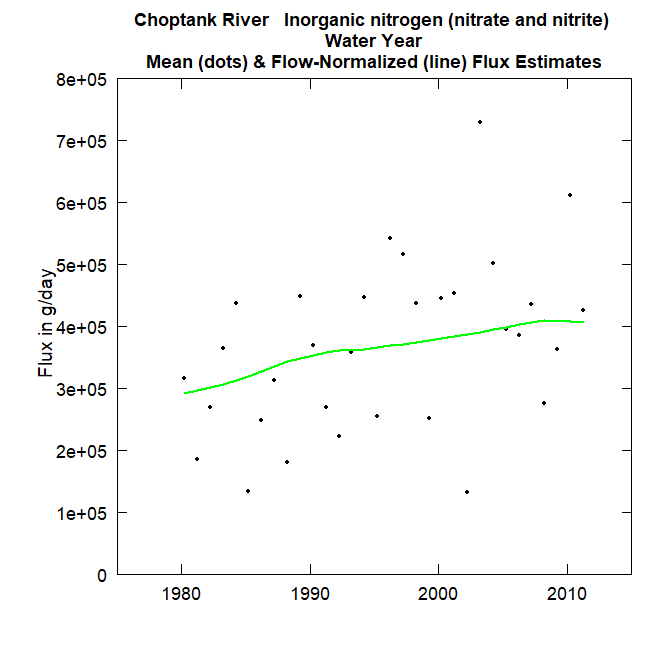# Month

There is also a way to customize the way the months are displayed. This might be useful for non-English speaking users for instance. Although we don’t have the capacity to have a conversion for all of the text, this could come in handy:

spanish_month <- new("monthLabel",
monthAbbrev = c("enero",   "feb",  "marzo", "abr",
"mayo",    "jun",  "jul", "agosto", "set",
"oct", "nov", "dic"),
monthFull = c("enero", "febrero",  "marzo", "abril",
"mayo",  "junio",    "julio", "agosto", "septiembre",
"octubre",   "noviembre", "diciembre"),
monthSingle = c("E", "F", "M", "A", "M", "J", "J",
"A", "S", "O", "N", "D"))

eList$INFO$param.units <- "mg/L"

concentration_esp <- new("concUnit",
longPrefix = "Concentración",
shortPrefix = "conc.")

boxConcMonth(eList, printTitle = FALSE, showXLabels = FALSE,
monthLab = spanish_month, concLab = concentration_esp)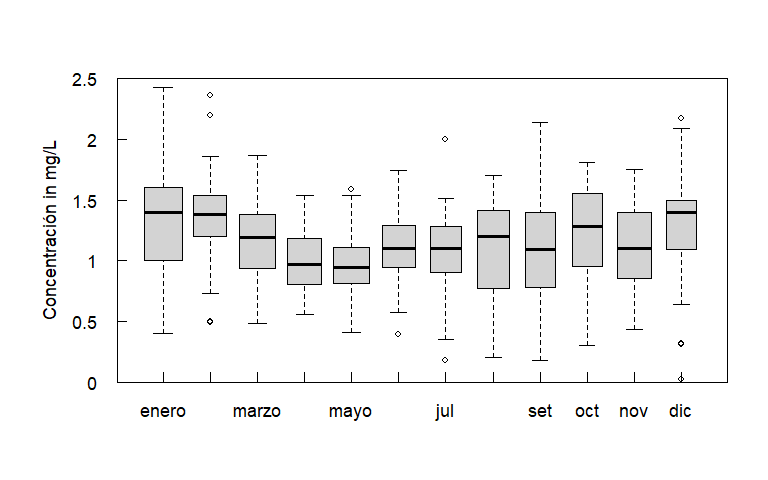Concentration by month.